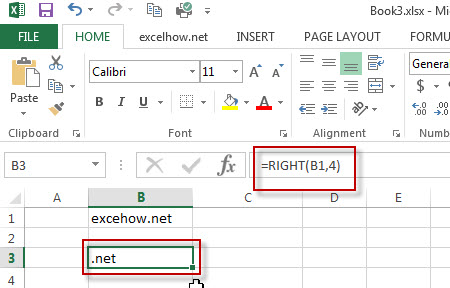# Excel Right Function

This post will guide you how to use Excel RIGHT function with syntax and examples in Microsoft excel.

### Description

The Excel RIGHT function returns a substring (a specified number of the characters) from a text string, starting from the rightmost character.

The RIGHT function is a build-in function in Microsoft Excel and it is categorized as a Text Function.

The RIGHT function is available in Excel 2016, Excel 2013, Excel 2010, Excel 2007, Excel 2003, Excel XP, Excel 2000, Excel 2011 for Mac.

### Syntax

The syntax of the RIGHT function is as below:

`= RIGHT (text,[num_chars])`

Where the RIGHT function arguments are:

• Text -This is a required argument. The text string that you want to extract from.
• num_chars-This is an optional argument. It will specify the number of characters that you want to extract from Text string.
Note: The Num_chars value must be greater than or equal to zero. If Num_chars is greather that the length of text string, it will return all of text string. If Num_chars value is omitted, it is set to be 1 by default.

### Example

The below examples will show you how to use Excel RIGHT Text function to extract the rightmost substring from a text string.

#1 To extract the rightmost 4 characters in the B1 cell, just using formula: =RIGHT(B1,4).### More RIGHT Function Examples

• Split Text and Numbers
If you want to split text and numbers from one cell into two different cells, you can use a formula based on the FIND function, the LEFT function or the RIGHT function and the MIN function. .…
• Highlight Cells That End with Specfic Characters
If you want to highlight cells that end with a specific text string, you can create a simple formula based on the COUNTIF function to apply conditional formatting. ……